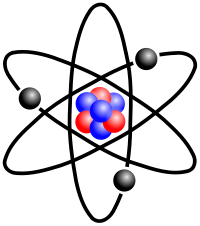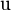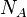# Atomic mass

"Atomic mass unit" redirects here. "Atomic mass" should not be confused with "atomic weight."A stylized lithium-7 atom, showing 3 protons, 4 neutrons, and 3 electrons (each electron is about 1800 times smaller than a proton or neutron). Rare Lithium-6 has only 3 neutrons, reducing the atomic weight (average) to 6.941.

The atomic mass (ma) is the mass of a single atom, when the atom is at rest at its lowest energy level (or "ground state"). Given that a chemical element may exist as various isotopes, possessing different numbers of neutrons in their atomic nuclei, atomic mass is calculated for each isotope separately. Atomic mass is most often expressed in unified atomic mass units, where one unified atomic mass unit is defined as one-twelfth the mass of a single atom of the carbon-12 isotope.

## Clarification of terminology

The atomic mass should be distinguished from other terms such as relative atomic mass and mass number.

• Relative atomic mass and atomic weight: The relative atomic mass (Ar) of an element is the ratio of the mass of an atom of the element to one-twelfth the mass of an atom of carbon-12. Because an element in nature is usually a mixture of isotopes, the relative atomic mass is also the weighted mean of the atomic masses of all the atoms in a particular sample of the element, weighted by isotopic abundance. In this sense, relative atomic mass was once known as atomic weight.
• Mass number: The mass number of an isotope is the total number of nucleons (neutrons plus protons) in the nucleus of each atom of the isotope. Rounding the atomic mass of an isotope usually gives the total nucleon count. The neutron count can then be derived by subtracting the atomic number (number of protons) from the mass number.

Often an element has one predominant isotope. In such a case, the actual numerical difference between the atomic mass of that main isotope and the relative atomic mass or standard atomic weight of the element can be very small, such that it does not affect most bulk calculations; but such an error can be critical when considering individual atoms. For elements with more than one common isotope, the difference between the atomic mass of the most common isotope and the relative atomic mass of the element can be as much as half a mass unit or more (as in the case of chlorine). The atomic mass of an uncommon isotope can differ from the relative atomic mass or standard atomic weight by several mass units.

An element may have different atomic weights depending on the source. Nevertheless, given the cost and difficulty of isotope analysis, it is usual to use the tabulated values of standard atomic weights, which are ubiquitous in chemical laboratories.

## Unified atomic mass unit

The unified atomic mass unit (u), or dalton (Da), or, sometimes, universal mass unit, is a unit of mass used to express atomic and molecular masses. It is defined as one-twelfth of the mass of an unbound atom of carbon-12 (12C) at rest and in its ground state.

1 u = 1/NA gram = 1/ (1000 NA) kg   (where NA is Avogadro's number)
1 u = 1.660538782(83)×10−27 kg = 931.494027(23) MeV/c2

The atomic mass unit (amu) is an older name for a very similar scale. The symbol amu for atomic mass unit is not a symbol for the unified atomic mass unit. It may be observed as an historical artifact, written during the time when the amu scale was used, or it may be used correctly when referring to its historical use. Occasionally, it may be used in error (possibly deriving from confusion about its historical usage).

The unified atomic mass unit, or dalton, is not an SI unit of mass, but it is accepted for use with SI under either name. Atomic masses are often written without any unit, and then the unified atomic mass unit is implied.

In biochemistry and molecular biology, when referring to macromolecules such as proteins or nucleic acids, the term "kilodalton" is used, with the symbol kDa. Because proteins are large molecules, their masses are given in kilodaltons, where one kilodalton is 1000 daltons.

The unified atomic mass unit is approximately equal to the mass of a hydrogen atom, a proton, or a neutron.

Technically, atomic mass is equal to the total mass of protons, neutrons, and electrons in the atom (when the atom is motionless), plus the mass contained in the binding energy of the atom's nucleus. However, the mass of an electron (being approximately 1/1836 of the mass of a proton) and the mass contained in nuclear binding (which is generally less than 0.01 u) may be considered negligible when compared with the masses of protons and neutrons. Thus, atomic mass is approximately equal to the total mass of the protons and neutrons in the nucleus of the atom. Thus, in general terms, an atom or molecule that contains n protons and neutrons will have a mass approximately equal to n u.

Chemical element masses, as expressed in u, would all be close to whole number values (within 2 percent and usually within 1 percent) were it not for the fact that atomic weights of chemical elements are averaged values of the various stable isotope masses in the abundances which they naturally occur. For example, chlorine has an atomic weight of 35.45 u because it is composed of 76 percent 35Cl (34.96 u) and 24 percent 37Cl (36.97 u).

Another reason for using the unified atomic mass unit is that it is experimentally much easier and more precise to compare masses of atoms and molecules (that is, determine relative masses) than to measure their absolute masses. Masses are compared with a mass spectrometer (see below).

### Measurement of atomic masses

Direct comparison and measurement of the masses of atoms is achieved by the technique known as mass spectrometry. The equation is:

mass contribution = (percent abundance) (mass)

## History

In the history of chemistry, the first scientists to determine atomic weights were John Dalton, between 1803 and 1805, and Jöns Jakob Berzelius, between 1808 and 1826. Atomic weight was originally defined relative to that of the lightest element, hydrogen, which was assigned the unit 1.00. In the 1860s, Stanislao Cannizzaro refined atomic weights by applying Avogadro's law (notably at the Karlsruhe Congress of 1860). He formulated a law to determine atomic weights of elements: The different quantities of the same element contained in different molecules are all whole multiples of the atomic weight. On that basis, he determined atomic weights and molecular weights by comparing the vapor density of a collection of gases with molecules containing one or more of the chemical element in question.

In the first half of the twentieth century, up until the 1960s, chemists and physicists used two different atomic mass scales. The chemists used a scale such that the natural mixture of oxygen isotopes had an atomic mass 16, while the physicists assigned the same number 16 to the atomic mass of the most common oxygen isotope (containing eight protons and eight neutrons). However, because oxygen-17 and oxygen-18 are also present in natural oxygen, this led to two different tables of atomic mass.

The unified atomic mass unit was adopted by the International Union of Pure and Applied Physics in 1960 and by the International Union of Pure and Applied Chemistry in 1961. Hence, before 1961 physicists as well as chemists used the symbol amu for their respective (and slightly different) atomic mass units. The accepted standard is now the unified atomic mass unit (symbol u).

Comparison of u with the physical and chemical amu scales:

1 u = 1.000 317 9 amu (physical scale) = 1.000 043 amu (chemical scale).

The unified scale based on carbon-12, 12C, met the physicists' need to base the scale on a pure isotope, while being numerically close to the old chemists' scale.

## Conversion factor between atomic mass units and grams

The standard scientific unit for dealing with atoms in macroscopic quantities is the mole (mol), which is defined arbitrarily as the amount of a substance with as many atoms or other units as there are in 12 grams of the carbon isotope C-12. The number of atoms in a mole is called Avogadro's number (NA), the value of which is approximately 6.022 × 1023 mol-1.

One mole of a substance always contains almost exactly the relative atomic mass or molar mass of that substance (which is the concept of molar mass), expressed in grams; however, this is almost never true for the atomic mass. For example, the standard atomic weight of iron is 55.847 g/mol, and therefore one mole of iron as commonly found on earth has a mass of 55.847 grams. The atomic mass of an 56Fe isotope is 55.935 u and one mole of 56Fe will in theory weigh 55.935g, but such amounts of the pure 56Fe isotope have never existed.

The formulaic conversion between atomic mass and SI mass in grams for a single atom is:$m_{\rm{u}}={m_{\rm{grams}} \over N_{A}}$

where$\rm{u}$ is the atomic mass unit and$N_A$ is Avogadro's number.

## Relationship between atomic and molecular masses

Similar definitions apply to molecules. One can compute the molecular mass of a compound by adding the atomic masses of its constituent atoms (nuclides). One can compute the molar mass of a compound by adding the relative atomic masses of the elements given in the chemical formula. In both cases the multiplicity of the atoms (the number of times it occurs) must be taken into account, usually by multiplication of each unique mass by its multiplicity.

## Mass defects in atomic masses

The pattern of the amounts by which the atomic masses deviate from their mass numbers is as follows: the deviation starts positive at hydrogen-1, becomes negative until a minimum is reached at iron-56, iron-58 and nickel-62, then increases to positive values in the heavy isotopes, with increasing atomic number. This equals to the following: nuclear fission in an element heavier than iron produces energy, and fission in any element lighter than iron requires energy. The opposite is true of nuclear fusion reactions: fusion in elements lighter than iron produces energy, and fusion in elements heavier than iron requires energy.